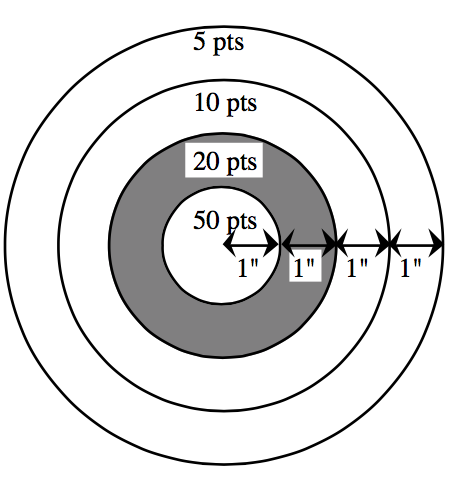### Home > CCA2 > Chapter C > Lesson C.1.1 > ProblemC-16

C-16.In one of the games at the County Fair, people pay to shoot a paint pistol at the target shown at right. The center has a radius of one inch. Each concentric circle has a radius one inch larger than the preceding circle. Assuming the paint pellet hits the target randomly, what is the probability that it hits:

1. The $50$-point ring?

Area of a circle $= πr^2$

$\text{P}(50\text{pt})\ = \frac{\text{area of success}}{\text{total area}}=\frac{\pi (1)^{2}}{\pi (4)^{2}}$

$\frac{1}{16}$

2. The $20$-point ring?

$\text{P}(20\text{pt})\ = \frac{\text{area of success}}{\text{total area}}=\frac{\pi (2)^{2}-\pi (1)^{2}}{\pi (4)^{2}}$